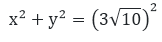×#### Thank you for registering.

One of our academic counsellors will contact you within 1 working day.

Click to Chat

1800-1023-196

+91-120-4616500

CART 0

• 0

MY CART (5)

Use Coupon: CART20 and get 20% off on all online Study Material

ITEM
DETAILS
MRP
DISCOUNT
FINAL PRICE
Total Price: Rs.

There are no items in this cart.
Continue Shopping• Complete JEE Main/Advanced Course and Test Series
• OFFERED PRICE: Rs. 15,900
• View Details

```Chapter 8: Quadratic Equations Exercise – 8.10

Question: 1

The hypotenuse of a right triangle is 25 cm. The difference between the lengths of the other two sides of the triangle is 5 cm. Find the lengths of these sides.

Solution:

Let the length of one side of the right triangle be x cm then,

the other side be = (x + 5) cm

and given that hypotenuse = 25 cm

By using Pythagoras Theorem,

x2 + (x + 5)2 = 252

x2 + x2 + 10x + 25 = 625

2x2 + 10x + 25 - 625 = 0

2x2 + 10x - 600 = 0

x2 + 5x - 300 = 0

x2 - 15x + 20x - 300 = 0

x(x - 15) + 20(x -15) = 0

(x - 15)(x + 20) = 0

x = 15  or x = - 20

Since, the side of triangle can never be negative

Therefore, when, x = 15

And, x + 5 = 15 + 5 = 20

Therefore, length of side of right triangle is = 15 cm and other side is = 20 cm

Question: 2

The diagonal of a rectangular field is 60 meters more than the shorter side. If the longer side is 30 meters more than the shorter side, find the sides of the field.

Solution:

Let the length of smaller side of rectangle be x metres then, the larger side be (x + 30) metres and

diagonal be = (x + 60) metres

By using Pythagoras theorem,

x2 + (x + 30)2 = (x + 60)2

x2 + x2 + 60x + 900 = x2 + 120x + 3600

2x2 + 60x + 900 - x2 - 120x - 3600 = 0

x2 - 60x - 2700 = 0

x2 - 90x + 30x - 2700 = 0

x(x - 90) + 30(x - 90) = 0

(x - 90)(x + 30) = 0

x = 90   or x = -30

Since, the side of rectangle can never be negative

Therefore, x = 90

x + 30 = 90 + 30 = 120

Therefore, the length of smaller side of rectangle is = 90 metres and larger side is = 120 metres.

Question: 3

The hypotenuse of a right triangle is 3√10 cm. If the smaller leg is tripled and the longer leg doubled, new hypotenuse will be 9√5 cm. How long are the legs of the triangle?

Solution:

Let the length of smaller side of right triangle be = x cm then large side be = y cm
By using Pythagoras theorem,x2 + y2 = 90  .... eqn. (1)

If the smaller side is triple and the larger side is doubled, the new hypotenuse is 9√5 cm

Therefore,9x2 + 4y2 = 405  .... eqn. (2)

From equation (1) we get,

y2 = 90 - x2

Now putting the value of y2 in eqn. (2)

9x2 + 4(90 - x2) = 405

9x2 + 360 - 4X2 - 405 = 0

5x2 - 45 = 0

5(x2 - 9) = 0

x2 - 9 = 0

x2 = 9

x = √9

x = ±3

Since, the side of triangle can never be negative

Therefore, when x = 3

Then, y2 = 90, x2 = 90 , (3)2 = 90, 9 = 81

y = √81

y = ±9

Hence, the length of smaller side of right triangle is = 3 cm and larger side is = 9 cm

Question: 4

A pole has to be erected at a point on the boundary of a circular park of diameter meters in such a way that the difference of its distances from two diametrically opposite fixed gates A and B on the boundary is 7 meters. Is it possible to do so? If yes, at what distances from the two gates should the pole be erected?

Solution:

Let P be the required location on the boundary of circular park such that its distance from the gate B is x metres that is BP = x metres

Then, AP = x + 7

In right triangle ABP, by using Pythagoras theorem,

AP2 + BP2 = AB2

(x + 7)2 + x2 = 132

x2 + 14x + 49 + x2 = 169

2x2 +14x + 49 - 169 = 0

2x2 + 14x - 120 = 0

2(x2 + 7x - 60) = 0

x2 + 12x - 5x - 60 = 0

x(x + 12) - 5(x + 12) = 0

(x + 12)(x - 5) = 0

x = - 12  or x = 5

Since, the side of triangle can never be negative

Therefore, P is at a distance of 5 metres from the gate B
```### Course Features

• 728 Video Lectures
• Revision Notes
• Previous Year Papers
• Mind Map
• Study Planner
• NCERT Solutions
• Discussion Forum
• Test paper with Video Solution